# Adding and Subtracting Decimals Lesson Plan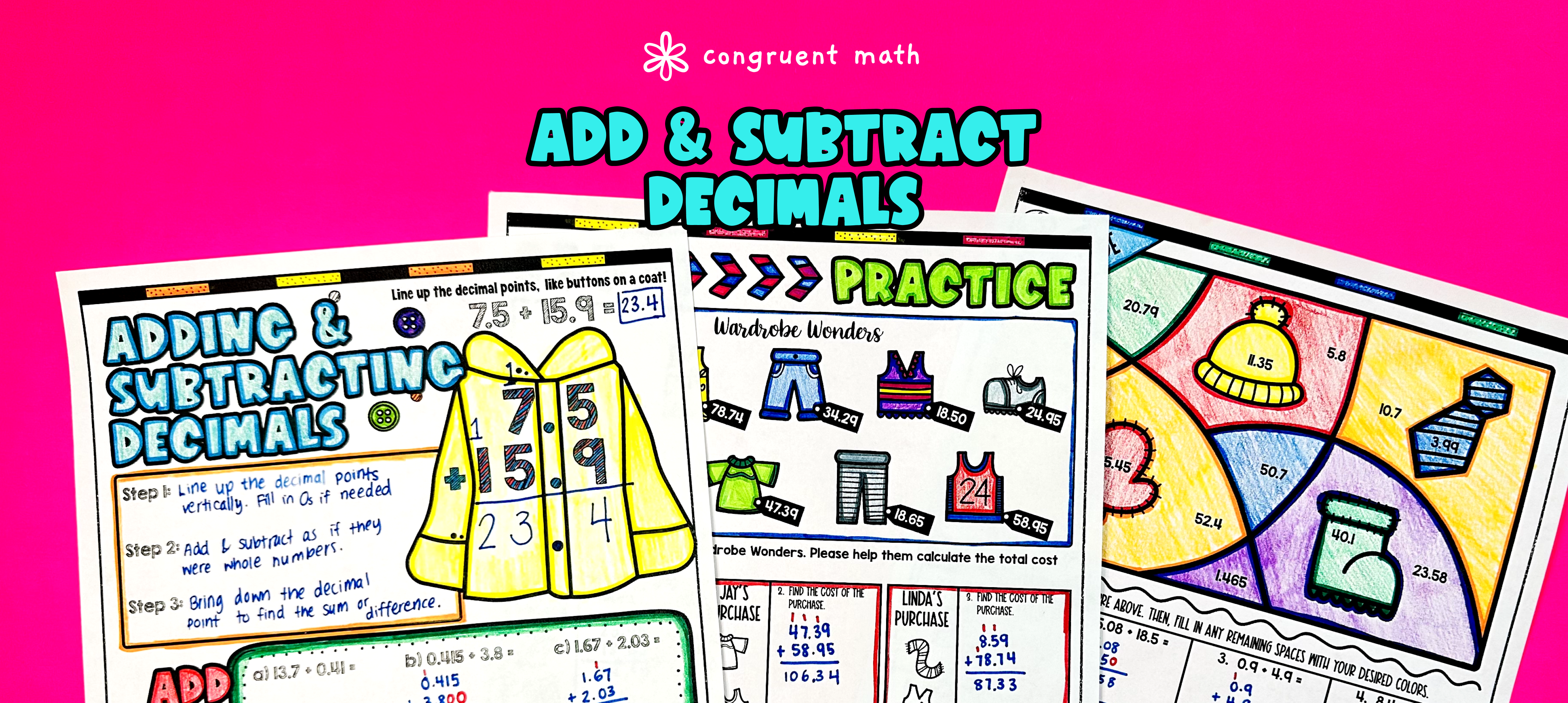Pin This

## Overview

Ever wondered how to teach adding and subtracting decimals in an engaging way to your 5th and 6th grade math students?

In this lesson plan, students will learn about decimal addition and subtraction and their real-life applications. Through artistic, interactive guided notes, check for understanding, a doodle and color by number activity, and a maze worksheet, students will gain a comprehensive understanding of adding and subtracting decimals.

The guided notes provide structured instruction steps to add and subtract decimals by lining up the decimal points, integrating checks for understanding to ensure students are on the right track. The practice worksheets, including a maze, word problems, and a color by code activity, allow students to apply their knowledge and reinforce their understanding of decimal addition and subtraction.

The lesson culminates with a real-life example that explores how decimal addition and subtraction can be applied to grocery shopping, giving students a clear understanding of how these skills are used in the real world.

By combining creativity, interactive activities, and real-life applications, this lesson plan provides an engaging and comprehensive introduction to adding and subtracting decimals for 5th and 6th grade math students.

## Get the Lesson Materials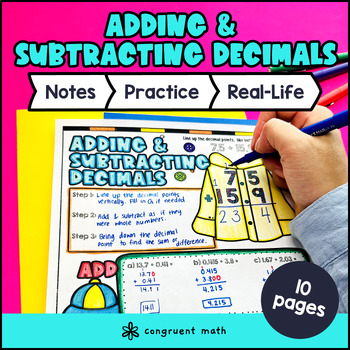\$4.25

## Learning Objectives

After this lesson, students will be able to:

• Solve word problems involving decimal addition and subtraction

• Apply the concept of adding and subtracting decimals to solve real-life problem

## Prerequisites

Before this lesson, students should be familiar with:

• Place value

• Basic addition and subtraction of whole numbers

• Decimals

• Sum

• Difference

## Procedure

### Introduction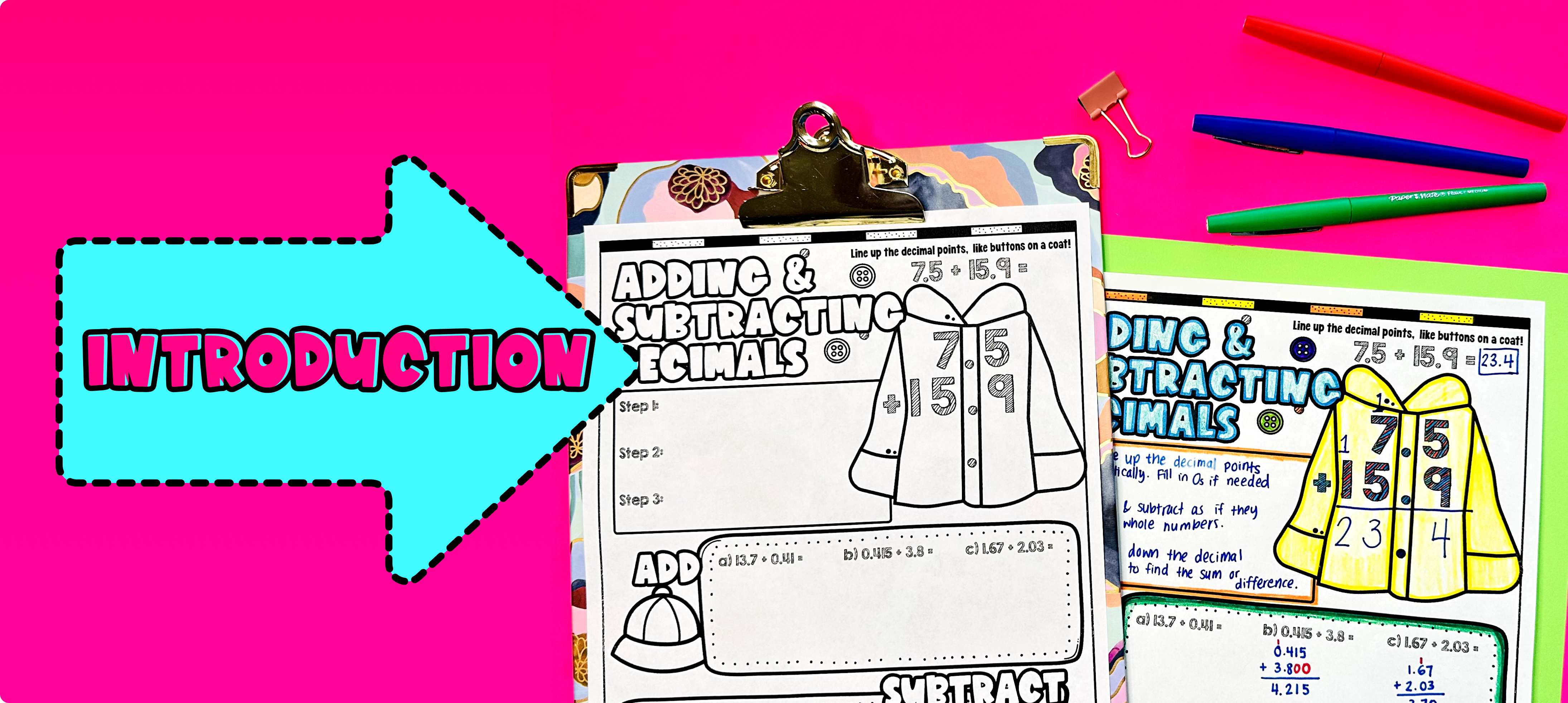Pin This

As a hook, ask students why understanding and being able to add and subtract decimals is important in real life. You can refer to the real-life application section on the last page of the guided notes for ideas. For example, you could ask them how adding and subtracting decimals can be useful when grocery shopping and calculating prices.

Use the guided notes to introduce the topic of adding and subtracting decimals. Walk through the key steps, such as the rules for adding and subtracting decimals, lining up decimal points, adding 0s to fill empty place values, and bringing decimal points down . Refer to the FAQ section below for a walk-through on this, as well as ideas on how to respond to common student questions.

Based on student responses during the discussion, reteach any concepts that students may need extra help with. If your class has a wide range of proficiency levels, you can pull out students for reteaching, while more advanced students can begin working on the practice exercises.

Note: The introduction section of the lesson plan is focused on engaging students and providing an overview of the topic. The specific details and steps for teaching the concept are included in the guided notes section of the lesson plan.

### Practice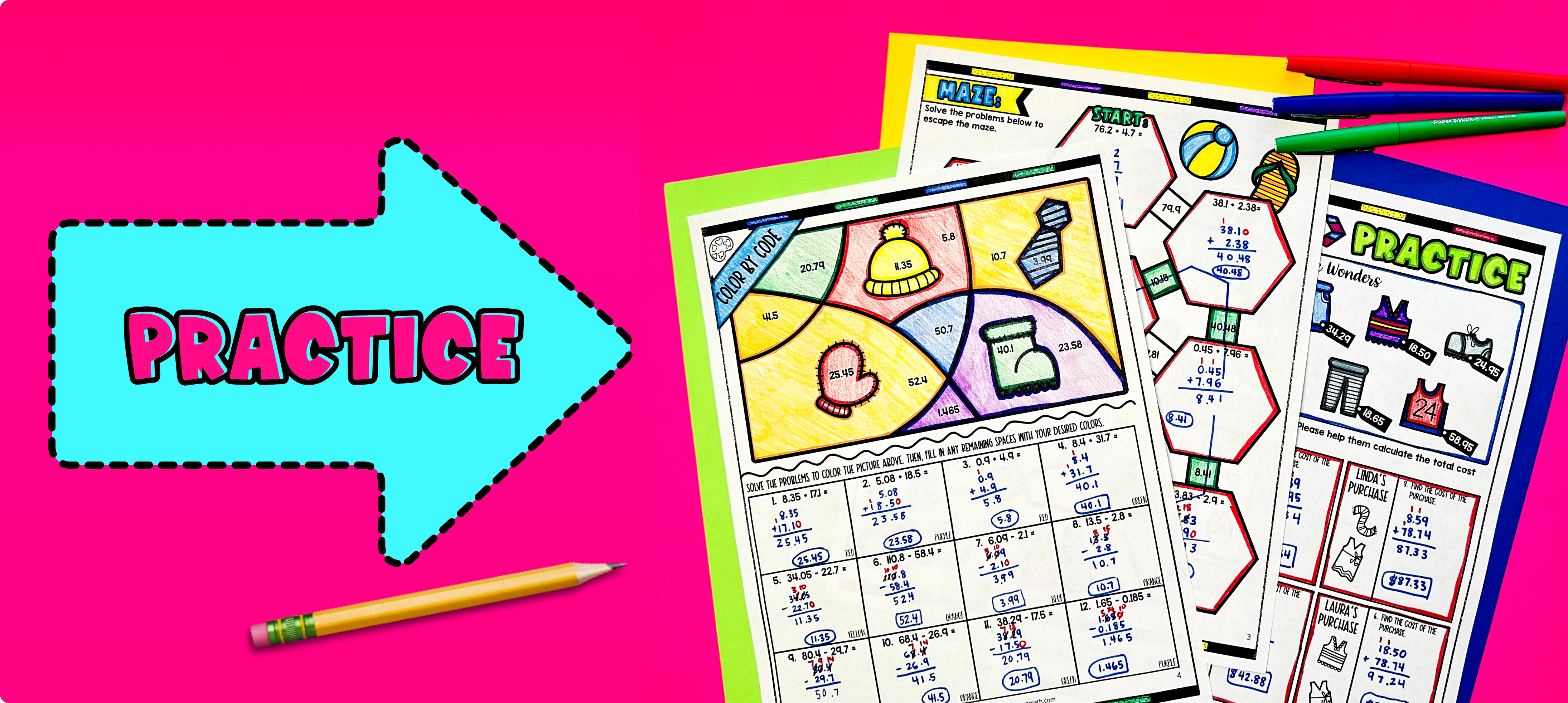Pin This

Have students practice word problems (they go on a clothes shopping spree!) by adding and subtracting decimals using the practice worksheets included in the resource. Walk around the classroom to answer any student questions and provide support as needed.

Fast finishers can dive into the maze worksheet and color by number activity for extra practice. You can assign it as homework for the remainder of the class or classwork for the next day.

### Real-Life Application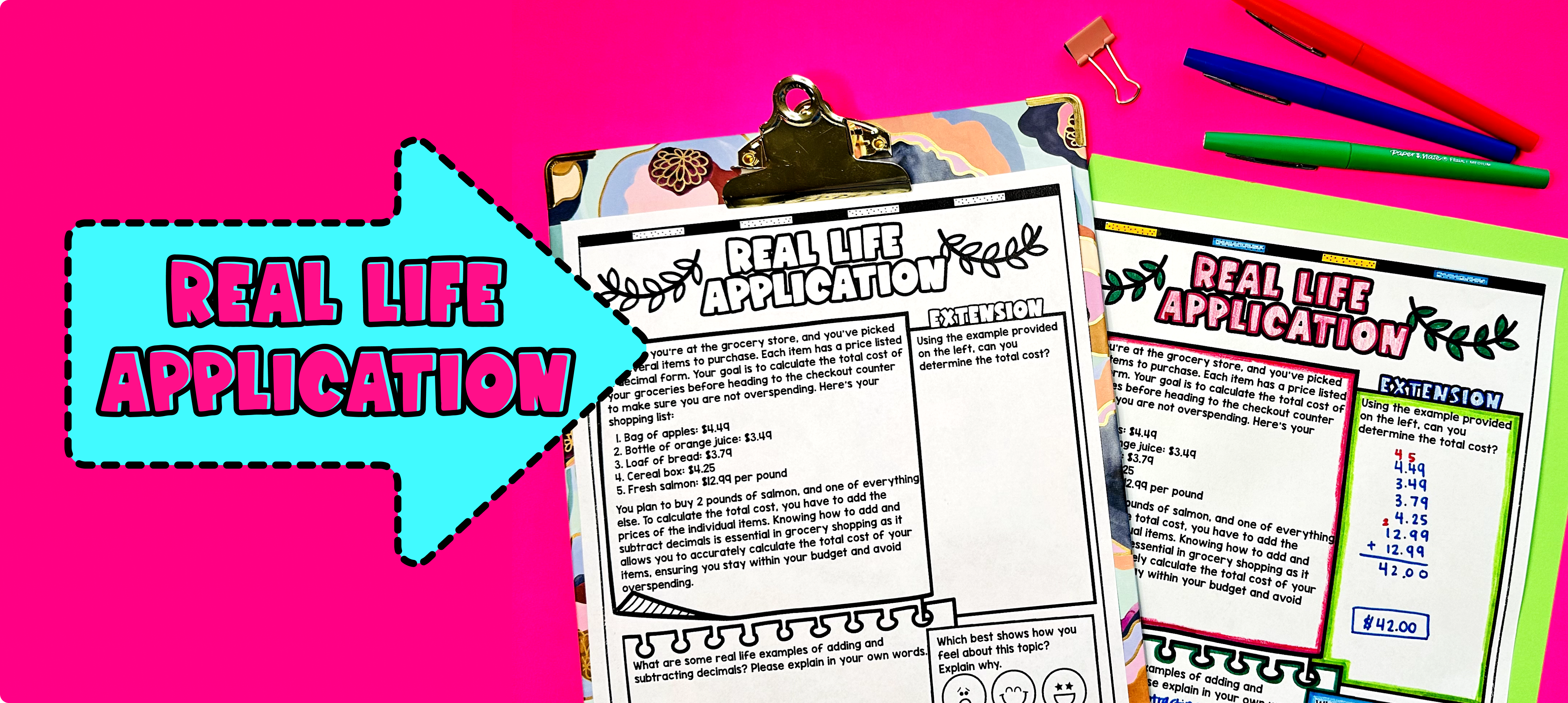Pin This

Bring the class back together, and introduce the concept of adding and subtracting decimals as applied to grocery shopping. Use the last page of the guided notes resource for this.

Explain to students that decimals are commonly used when calculating prices at the grocery store. Show them examples of grocery store receipts or advertisements that display prices with decimals.

Discuss with the students how decimals are used when adding up the total cost of items in a shopping cart. Emphasize the importance of understanding how to add and subtract decimals accurately to avoid overpaying or underpaying at the checkout counter.

Ask the students to imagine that they are shopping for groceries and have a budget of \$50. Distribute a list of grocery items along with their prices expressed in decimals. Instruct the students to choose items from the list, add up the prices, and determine if they can stay within their budget. Encourage them to use the skills they learned in the guided notes and practice worksheets to calculate the total cost accurately.

After they have completed the activity, have a class discussion about their experiences. Ask students to share how they made decisions about which items to purchase based on their budget. Discuss any challenges they faced when adding and subtracting decimals while shopping.

Refer to the FAQ for more ideas on how to teach real-world applications of adding and subtracting decimals.

## Extensions

If you’re looking for digital practice for adding and subtracting decimals, try the Pixel Art activities in Google Sheets. Every answer is automatically checked, and correct answers unlock parts of a mystery picture. It’s incredibly fun and a powerful tool for differentiation.

Here are some activities to explore:

A fun, no-prep way to practice adding and subtracting decimals is with Doodle Math - a fresh take on color by number or color by code. It includes multiple levels of practice, perfect for a review day or sub plan.

Here are some activities to try:

### Real-Life Math Project

A fun way to wrap up the lesson on adding and subtracting decimals is with a real-life math project. It enables students to see the application of the math in an engaging, extended project.

Here are some projects to consider:

## Want more ideas and freebies?

Get my free resource library with digital & print activities—plus tips over email.